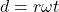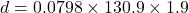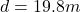Question

A disk 7.98 cm in radius rotates at a constant rate of 1 250 rev/min about its central axis. (a) Determine its angular speed. rad/s (b) Determine the tangential speed at a point 3.10 cm from its center. m/s (c) Determine the radial acceleration of a point on the rim. magnitude km/s2 direction (d) Determine the total distance a point on the rim moves in 1.90 s. m

1.We are given that

1 m=100 cm

a.Angular speed=1250rev/min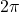rad=1 rev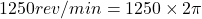rad/min

1 min=60 sec

Angular speed=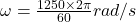Angular speed=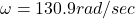b.r=3.1 cm=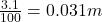1 m=100 cm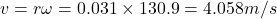Hence, the tangential speed=4.058m/s

c.Radial acceleration=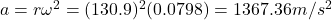Hence, the radial acceleration of a point on the rim=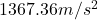d.Time, t=1.9 s

Total distance=